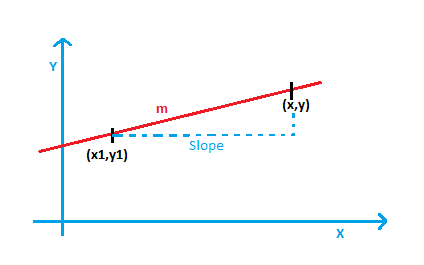# Equation of a Line Formula

An equation of a line can be expressed in many ways – Slope Intercept, Standard or Point-Slope. Here we will discuss the Point-Slope Equation of a Line.#### The Point Slope Equation of a Line Formula

$\LARGE y-y_{1}=m(x-x_{1})$

Where
m is the slope of the line.
x1 = x-coordinate of the point
y1 = y-coordinate of the point

### Solved Example

Question: Find the equation of the line whose slope is 8 and the coordinates of the point are (3, 5).

Solution:

Given, m = 8

(x1, y1) = (3, 5)

The formula for the equation of a line is,

y – y1 = m (x – x1)

y – 5 = 8 (x – 3)

y – 5 = 8x – 24

8x – y = 19

8x – y – 19 = 0

 More topics in Equation of a Line Formula Perpendicular Line Formula Parallel Line Formula Point of Intersection Formula